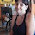## Sunday, September 20, 2015

### 14 911 923 | Terminator and Terminator 2 Foreshadowing, September 11 and September 23

As people well know, the number 911 was coded into the film Terminator 2.  Let us examine the date of September 11, to examine its curious numerology.

9/11/2001 = 9/(1+1)/(2+0+0+1) = 9/2/3
9+1+1+2+0+0+1 = 14

As it turns out, this number was also coded into Terminator 2 and Terminator, the original, from 1984.The only shot I noticed in Terminator 2 that has a connection to September 23

Notice Arnold's date of birth, July 30, 1947.  It has a connection to the year 'The Terminator' was released, 1984.

7+30+47 = 84

The film Terminator was released in the year '84.

10/26/1984 = 10+26+19+84 = 139 (Freemasonry = 139)
10/26/1984 = 1+0+2+6+1+9+8+4 = 31
10/26/1984 = 10+26+(1+9+8+4) = 58 (Destruction = 59)
10/26/84 = 10+26+84 = 120 (Terminator 2 released 7/3/1991 = 7+3+19+91 = 120)

With regards to the film coming out in the year '84', and the film seemingly foreshadowing the date of September 23, it is interesting to note that this year, on September 23, two important men with major '84' connections will be meeting in '84-land', the United States of America.  Those two men are of course, Pope Francis, the first Jesuit Pope, and Barack Obama, born 8/4, August 4.

Jesuit = 10+5+19+21+9+20 = 84
Obama = 50+2+1+30+1 = 84 (Jewish Gematria)

Obama is born August 4, 1961 and Pope Francis is born December 17, 1936.

8/4/1961
12/17/1936 = 12+17+19+36 = 84

United = 3+5+9+2+5+4 = 28
States = 1+2+1+2+5+1 = 12/21/30
of = 6+6 = 12
America = 1+4+5+9+9+3+1 = 32
United States of America = 84/93/102

Pope Francis and Barack Obama will be meeting at the White House on September 23, and White House has something in common with Terminator.

The film also features a good deal of '47' coding.

Sarah = 19+1+18+1+8 = 47
John = 10+15+8+14 = 47
TECH-NOIR = 2+5+3+8+5+6+9+9 = 47 (Where Sarah is first confronted by Terminator)
'47' was an important number in the World Trade Center attacks
Arnold was born in the year '47

The date of September 23, 2015 has a numerology of '47'.

9/23/15 = 9+23+15 = 47

Let us not forget the writer and director, James Cameron, born August 16, 1954.

James = 1+1+4+5+1 = 12/21
Cameron = 3+1+4+5+9+6+5 = 33
James Cameron = 45/54

August 16 is the 228th day of the year, which has a connection to United States of America, just as '84' does, the year of Terminator.

#### 1 comment:

1.The Life of an American Jew
in Racist Marxist Israel
Written in 1985 by Jack Bernstein
https://atrueott.wordpress.com/2012/10/02/world-war-iii-the-protocol-of-the-wise-men-of-zion/

That True Ott's blog. He's an ex Mormon who has a lot of interesting things to say about Zionist Jews from the Mormon's intel. Here's a Red Ice interview he gave.
Red Ice Radio - A. True Ott - Hour 1 - The World Power Structure